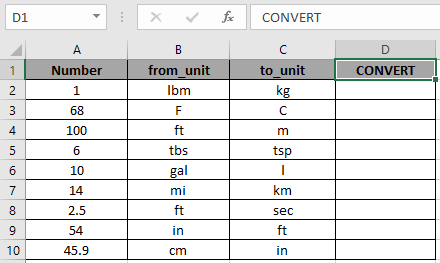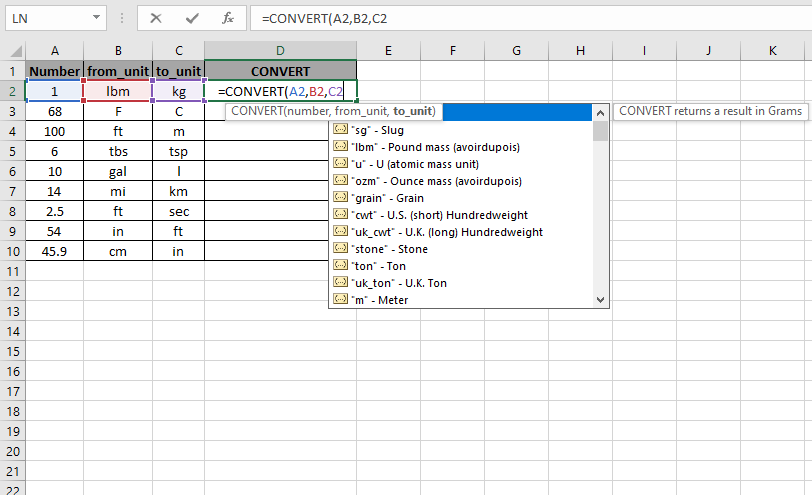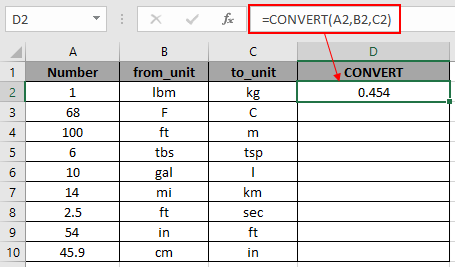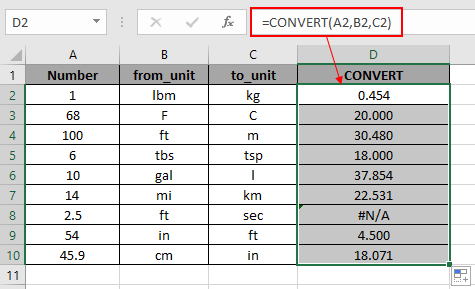# How to use the CONVERT Function in Excel

The CONVERT function converts the value of one measurement to another measurement. For example, weight measurements like pounds to gallons, length measurements like meters to feet or temperature measurements like Degree Celsius to Fahrenheit, etc.

Here are the types of measurements with their keywords which can be used to convert values.

Weight

 gram g slug sg Pound mass lbm U u ounce mass ozm grain grain U.S. hundredweight cwt or shweight Imperial hundredweight uk_cwt or lcwt stone stone ton ton Imperial ton uk_ton or LTON

Distance

 Meter m Statute mile mi Nautical mile nmi inch in foot ft yard yd Angstrom ang ell ell Light year ly Parsec pc pica pica

Time

 year yr day day hour hr minute mn or min second sec or s

Pressure

 Pascal pa Atmosphere atm mm of mercury mmHg PSI psi Torr Torr

Force

 Newton N Dyne dyn Pound force lbf Pond pond

Energy

 Joule J Erg e Thermodynamic calorie c IT calorie cal Electron - volt eV Horsepower - hour Hph Watt - hour Wh Foot - pound flb BTU BTU

Power

 Horsepower HP Pferdestärke PS Watt W

Temperature

 Degree Celsius C Degree Fahrenheit F Kelvin K Degree Rankine Rank Degree Réaumur Reau

Liquid Measure

 Teaspoon Tsp Modern Teaspoon tspm Cup cup U.S. pint pt U.K. pint uk_pt Quart qt Imperial quart uk_qt Gallon gal Imperial gallon uk_gal Litre l

Volume

 Cubic angstrom ang3 U.S. oil barrel barrel U.S. bushel bushel Cubic feet ft3 Cubic inch in3 Cubic light-year ly3 Cubic meter m3 Cubic Mile mi3 Cubic yard yd3 Cubic nautical mile nmi3 Cubic Pica pica3 Measurement ton (freight ton) MTON Gross Registered Ton GRT (regton)

Area

 International acre uk_acre statute acre us_acre Square angstrom and2 Are ar Square feet ft2 Hectare ha Square inches in2 Square light-year ly2 Square meters m2 Morgen morgen Square miles mi2 Square nautical miles nmi2 Square Pica pica2 Square yards yd2

Information

 Bit bit Byte byte

CONVERT function takes three arguments and returns the number after conversion to_unit mentioned as argument
Syntax:

=CONVERT ( number, from_unit, to_unit)

Number : number to convert
From_unit : unit of the number
To_unit : unit, number to be converted to

Let’s understand this function using it as an example.
Here we have a set of numbers in A2 : A10 and we need to convert the numbers from_unit to to_unit.For the first number, we need to convert the number from one pound mass to kilograms.

Use the formula:

= CONVERT ( A2, B2, C2 )Here all the arguments to the function are given as cell reference.

Press Enter to get the result.One pound mass is equal to 0.454 kilograms. See you didn't have to use your calculator for this and it's easy to use.

Now copy the formula to get different CONVERT values to the remaining cells and formula as shown in the snapshot below.As you can see from the outcomes in the snapshot above. The function returns an error in D8 cell as conversion between two different measurements is not possible.

Use the prefix measurements as shown in the table below.
Prefixes

 yotta Y zetta Z exa E peta P tera T giga G mega M kilo k hecto h dekao da deci d centi c milli m micro u nano n pico p femto f atto a zepto z yocto y

Use the above prefixes for accurate values.

Here are some of the observations gathered using the CONVERT function.

Note:

1. The CONVERT function returns #VALUE! Error if the data type argument is non -numeric.
2. The CONVERT function is case sensitive function.
3. The CONVERT function returns #NA error :
1. If the unit is invalid or doesn't exist.
2. If unit's prefix is invalid.
3. If the units belong to the different groups.

Hope you understood How to use the CONVERT function in Excel. Explore more articles on number conversion function here. Please state your query in the comment box below. We will assist you.

Related Articles

Convert from Stone to lbs

Convert minutes to hours and minutes

How to use Wildcards in Excel

Popular Articles

50 Excel Shortcut to Increase Your Productivity

Edit a dropdown list

If with conditional formatting

If with wildcards

Vlookup by date

Terms and Conditions of use

The applications/code on this site are distributed as is and without warranties or liability. In no event shall the owner of the copyrights, or the authors of the applications/code be liable for any loss of profit, any problems or any damage resulting from the use or evaluation of the applications/code.